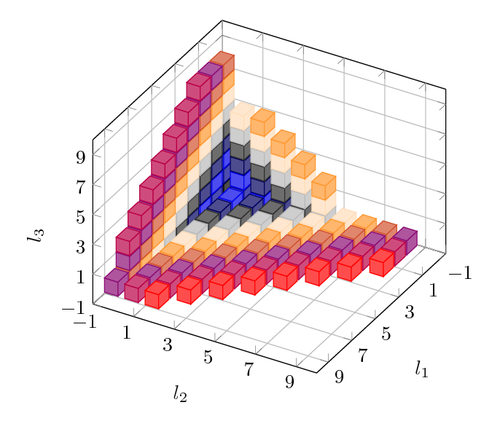# Solution to 35C3 Junior CTF challenge DANCEdDANCEd

crypto

500 (variable)

#### Writeup

This was the challenge description:

``````Sign up now, for the dance class you always wanted to visit! Totally secure, totally awesome! But be quick, the first few spots are already taken!

nc 35.207.159.114 1337

Source

Difficulty estimate: Medium
``````

And we also got the source for the server, written in Go, here.

The server logic is pretty straightforward. You log in to it using Telnet and can do two things that matter: either register yourself for a dance class, or list all the existing registrations which are encrypted.

Reading through the server code shows that for each encryption, a 64 byte “random” number is generated based on the number of previous registrations, 2 secret numbers and a lot of fixed values. That random number is then XORed to the combination of dance class and name. We guess that the flag is in the name of the first registrant.

To be able to decrypt the first registration, we first need to figure out the two secret numbers that are used to generate the 64 byte number. The generating function looks like this:

``````func doubleRound(block *uint32) {
for i := 0; i < 4; i++ {
block[(1+i)%4][i] = block[(1+i)%4][i] ^ bits.RotateLeft32(block[(0+i)%4][i]+block[(3+i)%4][i], 7)
block[(2+i)%4][i] = block[(2+i)%4][i] ^ bits.RotateLeft32(block[(1+i)%4][i]+block[(0+i)%4][i], 9)
block[(3+i)%4][i] = block[(3+i)%4][i] ^ bits.RotateLeft32(block[(2+i)%4][i]+block[(1+i)%4][i], 13)
block[(0+i)%4][i] = block[(0+i)%4][i] ^ bits.RotateLeft32(block[(3+i)%4][i]+block[(2+i)%4][i], 18)
}
for i := 0; i < 4; i++ {
block[i][(1+i)%4] = block[i][(1+i)%4] ^ bits.RotateLeft32(block[i][(0+i)%4]+block[i][(3+i)%4], 7)
block[i][(2+i)%4] = block[i][(2+i)%4] ^ bits.RotateLeft32(block[i][(1+i)%4]+block[i][(0+i)%4], 9)
block[i][(3+i)%4] = block[i][(3+i)%4] ^ bits.RotateLeft32(block[i][(2+i)%4]+block[i][(1+i)%4], 13)
block[i][(0+i)%4] = block[i][(0+i)%4] ^ bits.RotateLeft32(block[i][(3+i)%4]+block[i][(2+i)%4], 18)
}
}

func generateKeyStream(count uint32) []byte {
key := uint32{0xffffffff, 0xffffffff, 0xffffffff, 0xffffffff, 0xffffffff, 0xffffffff, 0xffffffff, 0xffffffff}
block := uint32{
{0x61707865, key, key, key},
{key, 0x3320646e, nonce, nonce},
{count, 0x00000000, 0x79622d32, key},
{key, key, key, 0x6b206574}}

for i := 0; i < 10; i++ {
doubleRound(&block)
}
for c := 0; c < 4; c++ {
for r := 0; r < 4; r++ {
fmt.Printf("%08x ", block[c][r])
}
fmt.Printf("\n")
}

var stream []byte
current := make([]byte, 4)
for c := 0; c < 4; c++ {
for r := 0; r < 4; r++ {
binary.LittleEndian.PutUint32(current, block[c][r])
stream = append(stream, current...)
}
}
return stream
}
``````

Analyzing the doubleRound() function shows that no information is lost in the transformations that happen, so it can easily be reversed like this:

``````func doubleRoundReverse(block *uint32) {
for i := 3; i >= 0; i-- {

block[i][(0+i)%4] = block[i][(0+i)%4] ^ bits.RotateLeft32(block[i][(3+i)%4]+block[i][(2+i)%4], 18)
block[i][(3+i)%4] = block[i][(3+i)%4] ^ bits.RotateLeft32(block[i][(2+i)%4]+block[i][(1+i)%4], 13)
block[i][(2+i)%4] = block[i][(2+i)%4] ^ bits.RotateLeft32(block[i][(1+i)%4]+block[i][(0+i)%4], 9)
block[i][(1+i)%4] = block[i][(1+i)%4] ^ bits.RotateLeft32(block[i][(0+i)%4]+block[i][(3+i)%4], 7)
}

for i := 3; i >= 0; i-- {

block[(0+i)%4][i] = block[(0+i)%4][i] ^ bits.RotateLeft32(block[(3+i)%4][i]+block[(2+i)%4][i], 18)
block[(3+i)%4][i] = block[(3+i)%4][i] ^ bits.RotateLeft32(block[(2+i)%4][i]+block[(1+i)%4][i], 13)
block[(2+i)%4][i] = block[(2+i)%4][i] ^ bits.RotateLeft32(block[(1+i)%4][i]+block[(0+i)%4][i], 9)
block[(1+i)%4][i] = block[(1+i)%4][i] ^ bits.RotateLeft32(block[(0+i)%4][i]+block[(3+i)%4][i], 7)
}
}
``````

Given this, we can perform the following steps to decrypt the flag.

• Make a reservation with a name consisting of 56 characters, to get 64 bytes of ciphertext
• XOR that ciphertext with the known plaintext, in order to get the generated key
• Run that key through our reversed function to unshuffle all the bits and get us the two secret numbers
• Use those two numbers and position number 0 to decrypt the first entry

This worked, and we got the flag: 35C3_DJ_B3RNSTE1N_IN_TH3_H0USE